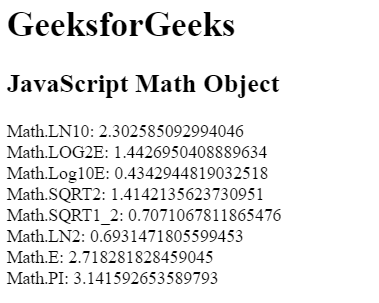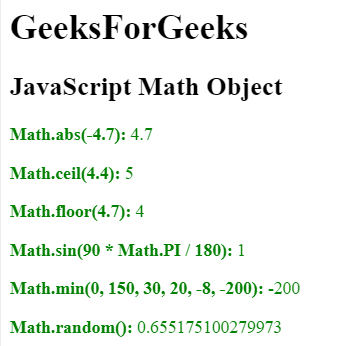# JavaScript | Math Object

The Math object is used to perform mathematical operations on numbers. There are many math object properties which are listed below:

Property Description
Math.E Euler’s number
Math.PI PI
Math.SQRT2 The square root of 2
Math.SQRT1_2 The square root of 1/2
Math.LN2 The natural logarithm of 2
Math.LN10 The natural logarithm of 10
Math.LOG2E Base 2 logarithm of E
Math.LOG10E Base 10 logarithm of E

Math objects: There are many math objects exists in JavaScript which are listed below:

Property Description
abs(x) Absolute value of x
acos(x) Arccosine of x, in radian
asin(x) Arcsine of x, in radian
atan(x) Arctangent of x, a numeric value between -PI/2 and PI/2 radian
atan2(y, x) Arctangent of the quotient of its arguments
ceil(x) Value of x rounded up to the nearest integer
cos(x) Cosine of x (x in radians)
exp() Value of E^x
floor() Value of x rounded below to the nearest integer
log() Natural logarithm (base E) of x
max(a, b, …) Highest value
min(a, b, …) Lowest value
pow(x, y) Value of x to power of y
random() Random number between 0 and 1
round(x) Value of x rounded to the nearest integer
sin(x) Sine of x (x in radians)
sqrt(x) Square root of x
tan(x) Tangent of angle

Example 1: This example use math object properties to return their values.

 `  ` `<``html``>  ` ` `  `<``head``>  ` `    ``<``title``>  ` `        ``JavaScript Math Object ` `    ``  ` `  ` `     `  `<``body``>  ` ` `  `    ``<``h1``>GeeksforGeeks  ` `         `  `    ``<``h2``>JavaScript Math Object  ` ` `  `    ``<``p` `id``=``"GFG"``> ` `     `  `    ```     `    ``<``script``>  ` `        ``document.getElementById("GFG").innerHTML =  ` `            ``"Math.LN10: " + Math.LN10 + "<``br``>" + ` `            ``"Math.LOG2E: " + Math.LOG2E + "<``br``>" + ` `            ``"Math.Log10E: " + Math.LOG10E + "<``br``>" + ` `            ``"Math.SQRT2: " + Math.SQRT2 + "<``br``>" + ` `            ``"Math.SQRT1_2: " + Math.SQRT1_2 + "<``br``>" + ` `            ``"Math.LN2: " + Math.LN2 + "<``br``>" + ` `            ``"Math.E: " + Math.E + "<``br``>" + ` `            ``"Math.PI: " + Math.PI; ` `    ``  ` `  ` ` `  `                     `

Output:Example 2: Math object methods are used in this example.

 `  ` `<``html``>  ` ` `  `<``head``>  ` `    ``<``title``>  ` `        ``JavaScript Math Object ` `    ``  ` `  ` `     `  `<``body``>  ` ` `  `    ``<``h1``>GeeksForGeeks  ` `         `  `    ``<``h2``>JavaScript Math Object  ` ` `  `    ``<``p` `id``=``"GFG"` `style` `= ``"color:green;"``> ` `     `  `    ```     `    ``<``script``>  ` `        ``document.getElementById("GFG").innerHTML =  ` `        ``"<``p``><``b``>Math.abs(-4.7): " + Math.abs(-4.7) + "" + ` `        ``"<``p``><``b``>Math.ceil(4.4): " + Math.ceil(4.4) + "" + ` `        ``"<``p``><``b``>Math.floor(4.7): " + Math.floor(4.7) + "" + ` `        ``"<``p``><``b``>Math.sin(90 * Math.PI / 180): " +  ` `                ``Math.sin(90 * Math.PI / 180) + "" + ` `        ``"<``p``><``b``>Math.min(0, 150, 30, 20, -8, -200): " + ` `                ``Math.min(0, 150, 30, 20, -8, -200) + "" + ` `        ``"<``p``><``b``>Math.random(): " + Math.random() + ""; ` `    ``  ` `  ` ` `  `                     `

Output:My Personal Notes arrow_drop_upCheck out this Author's contributed articles.

If you like GeeksforGeeks and would like to contribute, you can also write an article using contribute.geeksforgeeks.org or mail your article to contribute@geeksforgeeks.org. See your article appearing on the GeeksforGeeks main page and help other Geeks.

Please Improve this article if you find anything incorrect by clicking on the "Improve Article" button below.

Article Tags :

Be the First to upvote.

Please write to us at contribute@geeksforgeeks.org to report any issue with the above content.